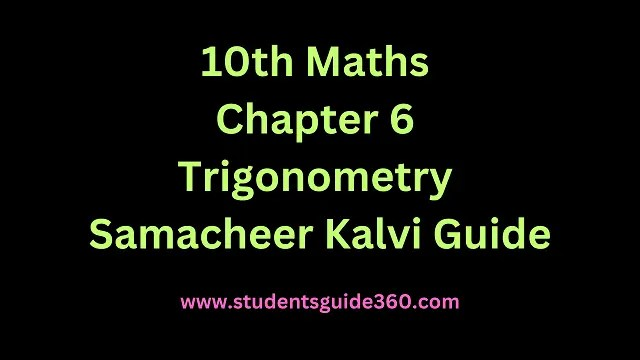# 10th Maths Chapter 6 Trigonometry Exercise 6.4

10th Standard Maths Chapter 6 Trigonometry Exercise 6.4 Guide. TN SSLC Samacheer Kalvi Guide Chapter 6 Exercise 6.4 Book Back Answers & Solutions. 10th All Subject Guide – Click Here. Class 1 to 12 All Subject Book Back Answers – Click Here.### 1. From the top of a tree of a height of 13 m the angle of elevation and depression of the top and bottom of another tree is 45° and 30° respectively. Find the height of the second tree. (√3 = 1.732)

Solution:### 2. A man is standing on the deck of a ship, which is 40 m above water level. He observes the angle of elevation of the top of a hill as 60° and the angle of depression of the base of the hill as 30°. Calculate the distance of the hill from the ship and the height of the hill. (√3 = 1.732)

Solution:∴ The height of the hill = 120 + 40 = 160 m
The distance of the hill from the ship is AC = x = 403–√ m = 69.28 m

### 3. If the angle of elevation of a cloud from a point ‘h’ meters above a lake is θ1 and the angle of depression of its reflection in the lake is θ2. Prove that the height that the cloud is located from the ground is h(tanθ1+tanθ2)/tanθ2−tanθ1

Solution:Let AB be the surface of the lake and let p be the point of observation such that AP = h meters.
Let C be the position of the cloud and C’ be its reflection in the lake. Then CB = C’B.
Let PM be ⊥r from P on CB
Then ∠CPM = θ1, and ∠MPC = θ2
Let CM = x.
Then CB = CM + MB = CM + PA
= x + hHence proved.

### 4. The angle of elevation of the top of a cell phone tower from the foot of a high apartment is 60° and the angle of depression of the foot of the tower from the top of the apartment is 30°. If the height of the apartment is 50 m, find the height of the cell phone tower. According to radiations control norms, the minimum height of a cell phone tower should be 120 m. State if the height of the above-mentioned cell phone tower meets the radiation norms.

Solution:Since 150m > 120m, yes the height of the above-mentioned tower meets the radiation norms.

### 5. The angles of elevation and depression of the top and bottom of a lamp post from the top of a 66 m high apartment are 60° and 30° respectively. Find

• (i) The height of the lamp post.
• (ii) The difference between the height of the lamp post and the apartment.
• (iii) The distance between the lamp post and the apartment. (3–√ = 1.732)

Solution:= 114.312 m.

### 6. Three villagers A, B, and C can see each T other across a valley. The horizontal distance between A and B is 8 km and the horizontal distance between B and C is 12 km. The angle of depression of B from A is 20° and the angle of elevation of C from B is 30°. Calculate :• (i) the vertical height between A and B.
• (ii) the vertical height between B and C. (tan20° = 0.3640, √3 = 1.732)

Solution: